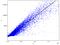# Introduction to Exploratory Data Analysis (EDA)

To share my understanding of the concept and techniques I know, I’ll take an example of House Prices dataset which is available on Kaggle and try to catch hold of as many insights from the data set using EDA.

Here is a quick overview of the things that you are going to learn in this article:

• Outlier Treatment
• Grouping of Data
• Handling missing values in dataset
• Correlation
`import pandas as pdimport numpy as npimport seaborn as snsimport matplotlib.pyplot as pltimport scipy.stats as stats `

## Descriptive Statistics

Descriptive Statistics helps to describe the basic features of dataset and…

# SQL Approach to Perform Data Analysis and Data Science Part-2

Basics of SQL is mentioned in Part-1 of SQL Approach.

In this article we will be covering various types of Joins and Subqueries

We will be working with Hr schema to demonstrate examples.

Multiple Table Queries

JOINS Clause is used to join two or more table, bases on a related column between different tables.

Types of Joins

1. Natural Joins
2. Equi Joins
3. Non Equi Join
4. Self Join
5. Left & Right Join
6. Inner & Outer Join

## Simple Join

SELECT t1. column_n, t2.columns_n ,….

FROM table_1 as t1

JOIN table_2 as t2

ON t1.column_n = t2.column_n;

# What is SQL ?

SQL stands for structured query language A query language is a sort of programming language designed to facilitate retrieving specific information from databases .

• Each column in a table is know as attribute and each row in table is know as record/tuple.

SQL can be devided 5 broad categories as follows;

Data Definition Language (DDL)

Data Manipulation Language (DML)

Data Query Language (DQL)

Data Control Language (DCL)

Transactional Control Language (TCL)

We will mainly be focusing…

# What is Linear Regression?

Let’s start with basics and define What regression is? Regression can be defined as a method used to determine the strength and character of relationship between one dependent variable (y) and some other variable known as independent variable (x).

When there’s a single independent variable (x), the method is referred to as simple linear regression. when there are multiple independent variables this method is known as multi linear regression.

The general form of Linear Regression model is:

y = m₁x₁ + m₂x₂ + m₃x₃ + . . . . . + mnxn + c + eLinear Regression## Vervit Khandelwal

Aspiring Data Scientist Linkedin: https://www.linkedin.com/in/vervit-khandelwal/